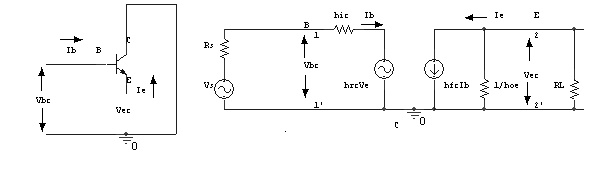Home | | Electronic Circuits II | Important Short Questions and Answers: Amplifier Circuits

# Important Short Questions and Answers: Amplifier Circuits

Electronic Devices and Circuits - Amplifiers - Important Short Questions and Answers: Amplifier

AMPLIFIERS

1. What is an amplifier?

An amplifier is a circuit, which can be used to increase the amplitude of the input current or voltage at the output by means of energy drawn from an external source.

2. Based on the transistor configuration how amplifiers are classified.

Based on transistor configuration, the amplifier are classified as

·                                         Common Emitter amplifier

·                                         Common Collector amplifier

·                                         Common Base amplifier

3. Draw a CE amplifier & its hybrid equivalent circuit.4. Draw a CC amplifier & its hybrid equivalent circuit.5. Write the Hybrid parameters equation for transistor amplifier?

Vi = hi Ii + hrVo

Io = hf Ii + hoVo

6.      Draw a CB amplifier & its hybrid equivalent circuit7. Which amplifier is called as voltage follower? Why?

The common collector transistor amplifier configuration is called as voltage follower. Since it has unity voltage gain and because of its very high input impedance.

It doesn’t draw any input current from the signal. So, the input signal is coupled to the output circuit without making any distortion.

8. Write the input impedance, output impedance, voltage gain and current gain of the common emitter amplifier in terms of h parameters for the fixed bias condition?

Current gain Ai = -hfe

Voltage gain Av = (hfeRC)/hie Input Impedance Zi = hie

Output Impedance Zo = RL ||RC

9. What are the limitations of h parameters?

The h parameters has the following limitations,

The accurate calculation of h parameters is difficult.

A transistor behaves as a two port network for small signals only, hence h parameters can be used to analyze only the small signal amplifiers.

10. Why hybrid parameters are called so? Define them?

The dimensions of the hybrid parameters are not alike, that is they are hybrid in nature so they are called hybrid parameters.

h11 = [ V1/I1] at V2=0; h11 = Inpu,t impedance with output port short circuited.

h12 = [ V1/V2] at I1=0; h12 = Reverse voltage gain with input port open circuited.

h21 = [ I2/I1] at V2=0; h11 = Forward current gain with output port short circuited.

h22 = [I2/V2] at I1=0; h11 = output impedance with input port open circuited.

11. What is transresistance amplifier?

In voltage shunt feedback amplifier the sampled signal is a voltage and the feedback signal (Which is fed in shunt) is a current.

Rm = Vo / Ii (or) Vo = Rm. Ii Where, Rm = Amplifier gain.

Vo = Output voltage. Ii = Input current.

12. What does bootstrapping mean? Why bootstrapping is done in a buffer amplifier?

In the emitter follower amplifier AV tends to unity. If a resistor is connected between input and output of the emitter follower, the change in the voltage at one end of the resistor changes the voltage at the other end of the resistor by same value. It is as if resistor is pulling itself up by its bootstraps. Such effect is known as boot strapping.

13. Draw the Darlington emitter follower circuit.14. How can a DC equivalent circuit of an amplifier be obtained?

The analysis of transistor circuits for small signal behaviour can be made by following simple guidelines. These guidelines are,

·                 Draw the actual circuit diagram

·                 Replace coupling capacitors and emitter bypass capacitor by short circuit

·                 Replace D.C. source by a short circuit

·                 Mark the points B, E, C on the circuit diagram and locate these points as the start of the equivalent circuit

·                 Replace the transistor by its h-parameter model

15.            State Miller’s Theorem.

It states that the effect of resistance Z on the input circuit is a ratio of input voltage to the current which flows from the input to the output.

Z1 = Z/(1-K)

It states that the effect of resistance Z on the output circuit is the ratio of output voltage to the current which flows from the output to input.

Z2 = Z-K / (K-1)

Study Material, Lecturing Notes, Assignment, Reference, Wiki description explanation, brief detail
Electronic Devices and Circuits : Amplifiers : Important Short Questions and Answers: Amplifier Circuits |